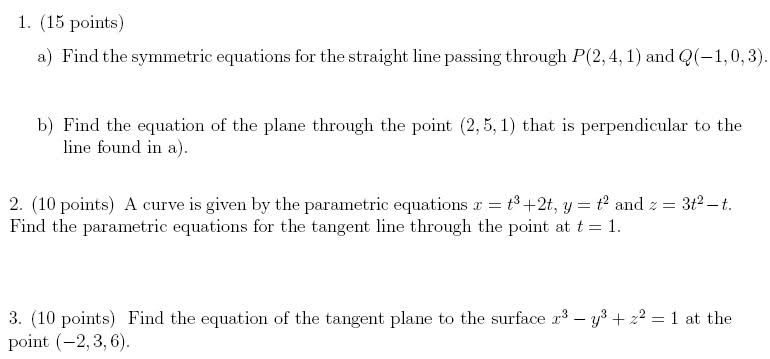# Calculus 3 problems (equations of planes and lines) 3 Space

Hi, Im currently doing a practice test for my final exam coming up, im wondering anyone can double check the questions to see if i did them write, below is a picture of the questions, the answers i got are listed at the bottom,

If you could, please post whether you agree with my answers to any of the following or if you disagree.## Homework Statement

for 1 A) i got, (X-2)/-3=(Y-4)/-4=(Z-1)/2

for 1 B) after simplifying i came up with -3x-4y+2z=-24

For 2) i got the following,

x= 3+5t
y=1+2t
z=2+5t

for 3) after simplifying i got, 3x-2y-5z=-42

## The Attempt at a Solution

HallsofIvy
Homework Helper
Hi, Im currently doing a practice test for my final exam coming up, im wondering anyone can double check the questions to see if i did them write, below is a picture of the questions, the answers i got are listed at the bottom,

If you could, please post whether you agree with my answers to any of the following or if you disagree.## Homework Statement

for 1 A) i got, (X-2)/-3=(Y-4)/-4=(Z-1)/2
If x= 2, y= 4, and z= 1, your equation becomes (2- 2)/(-3)= (4- 4)/(-4)= (1-1)/2 or 0= 0= 0 which is true. If x= -1, y= 0, and z= 3, your equation becomes (-1-2)/(-3)= (0-4)/(-4)= (3-1)/2 or 1= 1= 1 which is also true.

for 1 B) after simplifying i came up with -3x-4y+2z=-24
If x= 2, y= 5, and z= 1, this becomes -3(2)- 4(5)+ 2(1)= -6-20+ 2= -24. Yes, that point is in the plane. A vector perpendicular to this plane is <-2, -4, 2> which is the direction vector for the given line.

For 2) i got the following,

x= 3+5t
y=1+2t
z=2+5t
When t= 0 that gives (3,1,2) which is also the point given by t= 1 in the given parametric equations. Further, <5, 2, 5> is the direction vector and is the tangent vector to the given curve at t= 1.

for 3) after simplifying i got, 3x-2y-5z=-42
If x= -2, y= 3, and z= 6, your equation becomes 3(-2)- 2(3)- 5(6)= - 6- 6- 30= -42 so the point is on that plane. However, the normal vector to that plane is <3, -2, -5> while the the gradient of the given function is <3x^2, -3y^2, 2z> and at (-2, 3, 6), that is <12, -27, 12> which is NOT a multiple of <3, -2, -5> and so not in the same direction.

## The Attempt at a Solution

Do you agree with the answers i have?

Mark44
Mentor
HallsOfIvy disagrees with your answer to problem 3. Show us how you got the answer you've shown, and we'll figure it out.

HallsOfIvy disagrees with your answer to problem 3. Show us how you got the answer you've shown, and we'll figure it out.

I figured the problem i did wrong, i was thinking of a tangent plane to a line instead of a tangent plane to a surface, to fix it i ended up taking the gradient of the surface function, plugging in the point, then dot producting it with (x-xi),(y-yi),(z-zi)

i ended up with12x-27y+12z = -33

pretty sure this is right now

Mark44
Mentor
Looks fine.